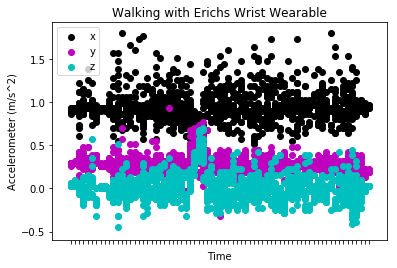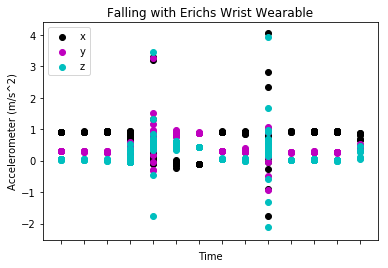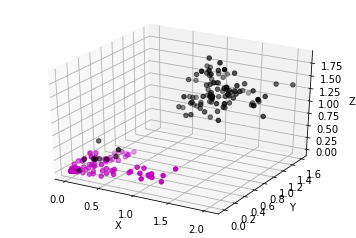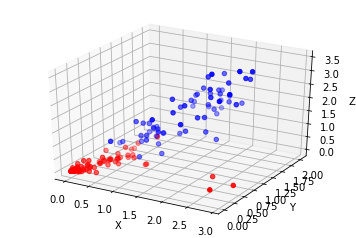# Motion Data Discovery¶

In this notebook, we will visualize the activities from two subjects wearing both wrist and waist wearables. The objective is to use the data from the wearables and create an accurate model for predicting a fall event.

This project is also part of my company, Symbiont Health, which strives to accelerate rescue and save lives through innovative fall detection systems.

## Preprocessing¶

Preprocessing included converting the JSON data format to CSV, adding the right labels to better naviagte the data, and slicing into strictly Erich's wrist data, Subject2's wrist data, Erich's waist data, etc... I would like to focus on the upcoming Support Vector Machine model as well the Long Short-Term Memory model, so I will just show a snippet of the preprocessed data.

The data is now becoming high dimensional as I have added max, min, mean, and variance columns for each x, y, z coordinate reading.

In :
import pandas as pd


   id  max_x  max_y  max_z  min_x  min_y  min_z    mean_x    mean_y    mean_z  \
0   1  1.376  0.480  0.160  0.608  0.064 -0.192  0.944410  0.302359  0.018051
1   2  1.376  0.480  0.576  0.736  0.064 -0.320  0.963404  0.317277 -0.012255
2   3  1.216  0.384  0.576  0.768  0.256 -0.320  0.920471  0.325647  0.015059
3   4  1.696  1.408  0.576 -0.416  0.224  0.000  0.842030  0.380179  0.138030
4   5  3.168  1.280  0.704 -0.384 -0.160 -0.320  0.982519  0.280296  0.046815

var_x     var_y     var_z          activity  isFall     timestamp  \
0  0.011835  0.005820  0.005214  Walk-Flat Ground       0  1.521639e+09
1  0.016699  0.006093  0.023511  Walk-Flat Ground       0  1.521639e+09
2  0.010906  0.002317  0.047841  Walk-Flat Ground       0  1.521639e+09
3  0.087581  0.066683  0.016054   Run-Flat Ground       0  1.521639e+09
4  0.300170  0.032007  0.013680              Jump       0  1.521639e+09

duration
0      4.58
1      4.98
2      4.10
3      4.94
4      4.98


## Visualization¶

### Walk Activity¶

The wearable data from walking shows that the x, y, z readings have low variance. This means that the $m/s^2$ accelerometer reading has low maximums (~+2$m/s^2$) and low minimums (~-0.5$m/s^2$).

In :
import matplotlib.pyplot as plt

# Creating a function that extracts the time data
def pullTime(event):
time_split = event.split(' ')
hours_minute = time_split[:8]
return hours_minute

Activities = 'Activities.csv'
# Establishing a column header for time
dataframe['time'] = dataframe.time.apply(pullTime)

# Providing a glimpse of the walking data

# Discovering the exact data that reflects my walking with the wrist wearable
Erich_wrist = dataframe.loc[dataframe.uid == 'a0:e6:f8:00:00:c0']
walk_flat_ground = Erich_wrist.loc[Erich_wrist.activity == 'Walk on flat ground']

fig,ax = plt.subplots(1)
plt.scatter(list(walk_flat_ground.time.values),list(walk_flat_ground.x.values),color='k',label='x')
plt.scatter(list(walk_flat_ground.time.values),list(walk_flat_ground.y.values),color='m',label='y')
plt.scatter(list(walk_flat_ground.time.values),list(walk_flat_ground.z.values),color='c',label='z')

plt.legend(loc='upper left')
plt.title('Walking with Erichs Wrist Wearable')
plt.xlabel('Time')
plt.ylabel('Accelerometer (m/s^2)')
ax.set_xticklabels([])
plt.show()

                 uid      x      y      z   timeStamp      time  \
0  a0:e6:f8:00:00:c2 -0.896 -0.288  0.096  1521638750  13:25:50
1  a0:e6:f8:00:00:c2 -0.928 -0.320  0.096  1521638750  13:25:50
2  a0:e6:f8:00:00:c2 -0.928 -0.288  0.096  1521638750  13:25:50
3  a0:e6:f8:00:00:c2 -0.896 -0.288  0.096  1521638750  13:25:50
4  a0:e6:f8:00:00:c2 -0.896 -0.320  0.096  1521638750  13:25:50

activity  activityID  isFall
0  Walk on flat ground           1       0
1  Walk on flat ground           1       0
2  Walk on flat ground           1       0
3  Walk on flat ground           1       0
4  Walk on flat ground           1       0### Fall Activity¶

The x, y, z accelerometer readings have quick and sudden changes as depicted in the scatterplot graph below. These extreme changes (~+4$m/s^2$ to ~-2$m/s^2$) are due to impact.

In :
# Snapshot of what the data looks like for a fall activity
print(dataframe[18008:18013])

# Defining fall data input from a singular individuals wrist wearable
fall = Erich_wrist.loc[Erich_wrist.activity == 'Fall']

fig,ax = plt.subplots(1)
plt.scatter(list(fall.time.values),list(fall.x.values),color='k',label='x')
plt.scatter(list(fall.time.values),list(fall.y.values),color='m',label='y')
plt.scatter(list(fall.time.values),list(fall.z.values),color='c',label='z')

plt.legend(loc='upper left')
plt.title('Falling with Erichs Wrist Wearable')
plt.xlabel('Time')
plt.ylabel('Accelerometer (m/s^2)')
ax.set_xticklabels([])
plt.show()

                     uid      x      y      z   timeStamp      time activity  \
18008  a0:e6:f8:00:00:c2 -0.928 -0.256  0.064  1521641513  14:11:53     Fall
18009  a0:e6:f8:00:00:c2 -0.896 -0.224  0.064  1521641513  14:11:53     Fall
18010  a0:e6:f8:00:00:c2 -0.928 -0.256  0.064  1521641513  14:11:53     Fall
18011  a0:e6:f8:00:00:c2 -0.928 -0.256  0.064  1521641513  14:11:53     Fall
18012  a0:e6:f8:00:00:c2 -0.896 -0.256  0.096  1521641513  14:11:53     Fall

activityID  isFall
18008          54       1
18009          54       1
18010          54       1
18011          54       1
18012          54       1### Falls vs Non-Falls¶

The 3D plot below shows falls in black and non-falls in pink. The recordings are from only Erich's wrist wearable. There is a difficult overlap of falls in the non-fall cluster, making many classification techniques, like k-NearestNeighbors and Guassian Mixture Model, unhelpful.

In :
import numpy as np
from mpl_toolkits.mplot3d import Axes3D

# isFall == 1 are recorded falls and vice versa. I am visualizing the variance between recordings.
fall_data = erich_wrist_data[erich_wrist_data['isFall'] == 1]
x_fall = np.array(fall_data['var_x'])
y_fall = np.array(fall_data['var_y'])
z_fall = np.array(fall_data['var_z'])

nonFall_data = erich_wrist_data[erich_wrist_data['isFall'] == 0]
x_nonFall = np.array(nonFall_data['var_x'])
y_nonFall = np.array(nonFall_data['var_y'])
z_nonFall = np.array(nonFall_data['var_z'])

axes = plt.subplot(111, projection='3d')
axes.scatter(x_fall, y_fall, z_fall, c='k')
axes.scatter(x_nonFall, y_nonFall, z_nonFall, c='m')

axes.set_zlabel('Z')
axes.set_ylabel('Y')
axes.set_xlabel('X')
plt.show()## Support Vector Machine (SVM)¶

This set of supervised learning is used for classification, regression, and outliers detection. They are also effective in high dimensional spaces. I am hoping to get a highly accurate model that can capture that chunk of black-colored falls that are clustered in our non-fall 3d area above.

I am also interesting in using three different kernel functions to test more svm methods. The kernel functions I use are:

• Linear <$x,x'$>
• Radial Basis Function (RBF) $exp(γ||x-x'||^2)$ γ must be greater than 0
• Sigmoid $tanh(γ<x,x'>+r)$ r is specified by coef0
In :
import random

wristSIZE = len(wristDAT)
waistSIZE = len(waistDAT)

# Split Wrist Data to Two Sets
trainDAT=list()
trainCLASS=list()
testDAT=list()
testCLASS=list()

for i in range(wristSIZE):
r = random.randint(0, 1)
wristBASE = list(wristDAT.iloc[i,1:13])
waistBASE = list(waistDAT.iloc[i,1:13])
if (r):
trainDAT.append(wristBASE + waistBASE)
trainCLASS.append(int(wristDAT.iloc[i,14:15]['isFall']))
else:
testDAT.append(wristBASE + waistBASE)
testCLASS.append(int(wristDAT.iloc[i,14:15]['isFall']))

from sklearn import svm

# Training the SVM Model
clfLIN = svm.SVC(kernel='linear').fit(trainDAT, trainCLASS)
clfRBF = svm.SVC(kernel='rbf').fit(trainDAT, trainCLASS)
clfSIG = svm.SVC(kernel='sigmoid').fit(trainDAT, trainCLASS)

# Testing the SVM Model
predLIN = clfLIN.predict(testDAT)
predRBF = clfRBF.predict(testDAT)
predSIG = clfSIG.predict(testDAT)

# Comparing the results for linear, rbf, and sigmoid
reltLIN = sum([1 for i,j in zip(testCLASS, predLIN) if int(i) == int(j)]) / len(testCLASS) * 100
reltRBF = sum([1 for i,j in zip(testCLASS, predRBF) if int(i) == int(j)]) / len(testCLASS) * 100
reltSIG = sum([1 for i,j in zip(testCLASS, predSIG) if int(i) == int(j)]) / len(testCLASS) * 100

print('The accuracy of the linear SVM model is: %.2f %%' % reltLIN)
print('The accuracy of the rbf SVM model is: %.2f %%' % reltRBF)
print('The accuracy of trhe sigmoid SVM model is: %.2f %%' % reltSIG)

The accuracy of the linear SVM model is: 96.67 %
The accuracy of the rbf SVM model is: 95.56 %
The accuracy of trhe sigmoid SVM model is: 96.67 %


### Results from 1st SVM¶

The above results are strong; however, they only apply to and SVM trained and tested on data from the two wearable on my own person. What if we were now to try training on my data and testing on subject2's data? In other words, how well would a model trained on a 6'0" 200lb male detect a 6'4" 170lb male's falls?

In :
## Load Bath Waist and Wrist Data
# combining the data of the waist and wrist sensor as train and test data set.
erich_waist_size = len(erich_waist_data)
erich_wrist_size = len(erich_wrist_data)

subject2_waist_size = len(subject2_waist_data)
subject2_wrist_size = len(subject2_wrist_data)

trainDAT=list()
trainCLASS=list()
for i in range(erich_waist_size):
base_waist_data = list(erich_waist_data.iloc[i,1:13])
base_wrist_data = list(erich_wrist_data.iloc[i,1:13])
isFall = int(erich_waist_data.iloc[i,14:15]['isFall'])

trainDAT.append(base_waist_data + base_wrist_data)
trainCLASS.append(isFall)

testDAT=list()
testCLASS=list()
for i in range(subject2_waist_size):
base_waist_data = list(subject2_waist_data.iloc[i,1:13])
base_wrist_data = list(subject2_wrist_data.iloc[i,1:13])
isFall = int(subject2_waist_data.iloc[i,14:15]['isFall'])

testDAT.append(base_waist_data + base_wrist_data)
testCLASS.append(isFall)

# Training the SVM Model
clfLIN = svm.SVC(kernel='linear').fit(trainDAT, trainCLASS)
clfRBF = svm.SVC(kernel='rbf').fit(trainDAT, trainCLASS)
clfSIG = svm.SVC(kernel='sigmoid').fit(trainDAT, trainCLASS)

# Testing the SVM Model
predLIN = clfLIN.predict(testDAT)
predRBF = clfRBF.predict(testDAT)
predSIG = clfSIG.predict(testDAT)

# Comparing the results for linear, rbf, and sigmoid
reltLIN = sum([1 for i,j in zip(testCLASS, predLIN) if int(i) == int(j)]) / len(testCLASS) * 100
reltRBF = sum([1 for i,j in zip(testCLASS, predRBF) if int(i) == int(j)]) / len(testCLASS) * 100
reltSIG = sum([1 for i,j in zip(testCLASS, predSIG) if int(i) == int(j)]) / len(testCLASS) * 100

print('The accuracy of the linear SVM model is: %.2f %%' % reltLIN)
print('The accuracy of the rbf SVM model is: %.2f %%' % reltRBF)
print('The accuracy of trhe sigmoid SVM model is: %.2f %%' % reltSIG)

The accuracy of the linear SVM model is: 79.80 %
The accuracy of the rbf SVM model is: 93.43 %
The accuracy of trhe sigmoid SVM model is: 45.96 %

In :
predDAT = predLIN

t_p = sum([1 for i,j in zip(testCLASS, predDAT) if int(i) == int(j) and int(i) == 1])
f_n = sum([1 for i,j in zip(testCLASS, predDAT) if int(i) != int(j) and int(i) == 1])
f_p = sum([1 for i,j in zip(testCLASS, predDAT) if int(i) != int(j) and int(i) == 0])
t_n = sum([1 for i,j in zip(testCLASS, predDAT) if int(i) == int(j) and int(i) == 0])

accuracy = (t_p + t_n) / (t_p + f_n + f_p + t_n)
average_accuracy = (t_p / (t_p + f_n) + t_n / (f_p + t_n)) / 2
precision = t_p / (t_p + f_p)
recall = t_p / (t_p + f_n)
f1_score = 2 * precision * recall / (precision + recall)
print(" Accuracy: %.2f\n Avg Accuracy %.2f\n Precision %.2f\n Recall %.2f\n F1-Score %.2f\n"
% (accuracy, average_accuracy, precision, recall, f1_score))

 Accuracy: 0.80
Avg Accuracy 0.73
Precision 0.81
Recall 0.52
F1-Score 0.63



### Results from 2nd SVM¶

The accuracy of the Support Vector Machine learning model using the Radial Basis Kernel Function is the most impressive result. The accuracy and scores above refelect that of the SVM using the basic linear kernel function.

NEXT: Visualization of Best Performer: Radial Basis Function

I would like to label the falls and nonfalls again black and pink, respectively. We can view how the radial basis function performed so well for this means of testing and training on different persons.

In :
labels = ['mean_x', 'mean_y', 'mean_z', 'max_x', 'max_y', 'max_z', 'min_x', 'min_y', 'min_z', 'var_x', 'var_y', 'var_z']
df1 = pd.DataFrame(testDAT, columns=labels)
df1['isFall'] = predRBF

labels2 = ['mean_x', 'mean_y', 'mean_z', 'max_x', 'max_y', 'max_z', 'min_x', 'min_y', 'min_z', 'var_x', 'var_y', 'var_z']
df2 = pd.DataFrame(testDAT, columns=labels2)
df2['isFall'] = testCLASS

fall_data = df1[df1['isFall'] == 1]
x_fall = np.array(fall_data['var_x'])
y_fall = np.array(fall_data['var_y'])
z_fall = np.array(fall_data['var_z'])

nonFALL_data = df2[df2['isFall'] == 0]
x_nonfall = np.array(nonFALL_data['var_x'])
y_nonfall = np.array(nonFALL_data['var_y'])
z_nonfall = np.array(nonFALL_data['var_z'])

axes = plt.subplot(111, projection='3d')
axes.scatter(x_fall, y_fall, z_fall, c='b')
axes.scatter(x_nonfall, y_nonfall, z_nonfall, c='r')

axes.set_zlabel('Z')
axes.set_ylabel('Y')
axes.set_xlabel('X')
plt.show()